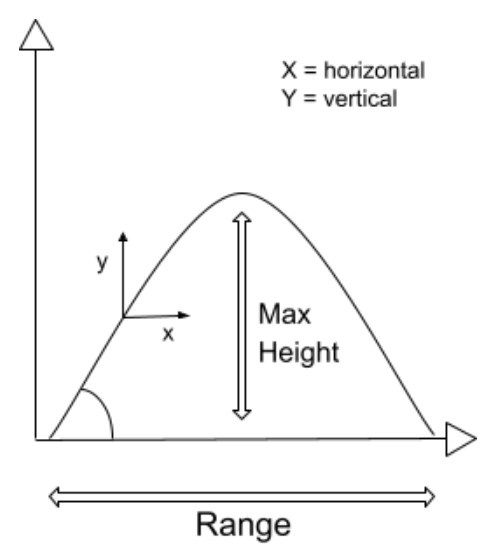# Vertical Velocity Calculator

To find the vertical velocity of projectile motion, enter the values of initial velocity, acceleration of gravity, and the time in the projectile motion calculator.

Formula:
vy = v0 - gt
m/s
m/s2
s

Give Us Feedback

Vertical velocity calculator finds the vertical velocity of an object moving in projectile motion.

## Projectile motion and its vertical factor

A projectile motion is such a motion in which a body moves along a parabolic trajectory.The velocity is divided into two components.

• Horizontal
• Vertical

The horizontal component of the velocity remains uniform but the vertical component changes with time.

Horizontal velocity is same as initial velocity throughout the motion. The vertical velocity changes by 9.8 m/s with every passing second.

## Formula of vertical velocity of projectile motion

The equation used to calculate the vertical velocity of projectile motion:

Vy = v0 - gt

In this equation:

v0 is initial velocity,

t is time, and

g is gravitational acceleration.

## How to calculate vertical velocity?

Example:

A football player kicked a ball into projectile motion. The ball lands on ground after 5 seconds. What will be the vertical component of velocity if initial velocity is 15m/s?

Solution:

Step 1: Write down the given values.

V0 = 15 m/s

t = 5 s

Gravitational acceleration= 9.8 ms-2

Step 2: Place the values in the formula.

Vy = v0 - gt

Vy = (15) - (9.8)(5)

Vy = -34 m/s

The negative sign represents downward motion.

### Math Tools